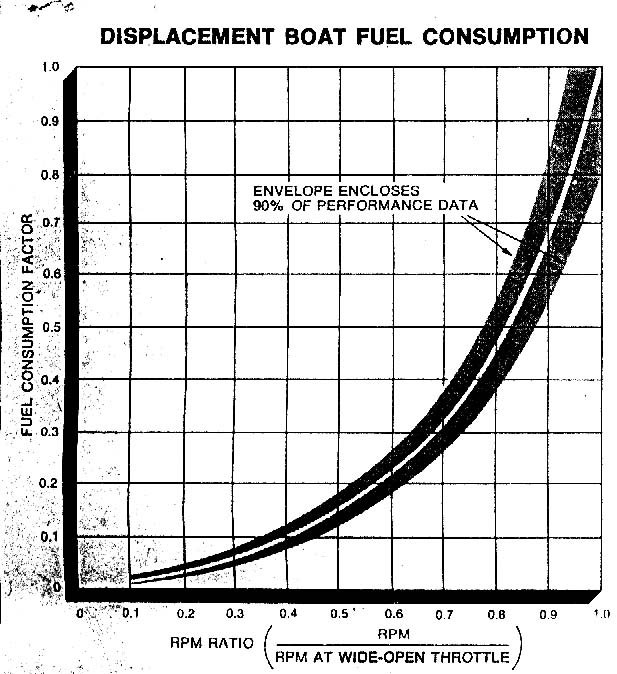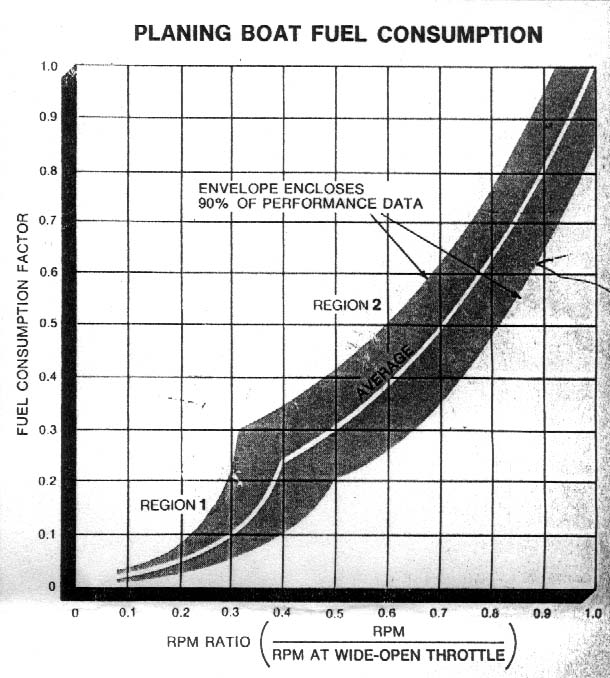## Fuel Efficient Cruising

These excerpts are taken from the April 1981 Motor Boat and Sailing article, "Fuel Efficient Cruising" by Mike Carlson. (click here for a PDF of the entire article).
Note: The article is in PDF format. If you don't have a PDF reader, go to www.adobe.com.

The basic formula for the GPH fuel consumption (gallons per hour) of your boat is found by this formula:

GPH = (Engine Horsepower) * (RPM at WOT) / 50,000.

For example, I have twin 135 HP, 2200 RPM at WOT (wide open throttle) so

GPH = (2*135) * 2200 / 50000

= 11.88 GPH

For turbo charged engines, use

GPH = (Engine Horsepower) * (RPM at WOT) / 45,000.
(Turbo owners: note the article states that this is less accurate for turbos!)

Now, for less than full throttle, Get the FCF (fuel consumption factor) from Figure 2 or 3 (depending on your type of boat) from the RPM Ratio of the desired RPM, then multiply this to the GPH. (If you read the article, you apply it to the full formula, but why recalculate the whole thing each time! So save time and do it my way!)

To continue my example, say I want to know GPH at 1800 RPM.

RPM Ratio = RPM / RPM at WOT

= 1800/2200

= .82

FCF @ .82 = 0.5

from graph on Figure 2 (I have a displacement boat)

GPH = (GPH@ WOT) * FCF

= 11.88 * 0.5

= 5.94 GPH @ 1800 RPM.

Another: at 1700 RPM, its ratio is .77, fcf is .42, so 4.98 GPH. Big savings for 100 RPM less!!!! I go only about 1/2 knot slower.

So, make yourself a graph of your GPH at various RPM's. You'll find that just a 20% reduction in throttle (from full) will result in a nearly 50% fuel savings! (see my example) Your graph should look just like either figure 2 or 3, meaning that your graph's curve should mimic the ones here. My graph can be seen here

Next, I will get the speed at each RPM, and then graph that. I think the most efficient RPM will be where the graphs cross. (to be completed!)Figure 2Figure 3
Region 1 is before you plane, then its Region 2.

Back to Knot Kidding